# Texas Go Math Grade 1 Lesson 8.4 Answer Key Solve Multi-Step Word Problems

Refer to our Texas Go Math Grade 1 Answer Key Pdf to score good marks in the exams. Test yourself by practicing the problems from Texas Go Math Grade 1 Lesson 8.4 Answer Key Solve Multi-Step Word Problems.

## Texas Go Math Grade 1 Lesson 8.4 Answer Key Solve Multi-Step Word Problems

Essential Question
How can you solve multi-step word problems?
Explanation:
First find the value which is used to find the question.

Explore
Use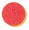or objects to model the problem. Draw theor objects. Write the numbers and the number sentences.

_______

_______
Explanation:
Kate had 7 birds and 4 were flied away. how many are with Kate now
7 – 4 = 3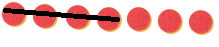Math Talk
Mathematical Processes
Explain the strategy you used to solve the multi-step problem. Is there another way to solve the problem?

For The Teacher

• Read the following problem. There are 11 crayons in the box. Pete takes 5 crayons. Jem takes 3 crayons. How many crayons are in the box?
Explanation:
first we have to add then to subtract
5 + 3 = 8
11 – 8 = 3
3 Crayons are in the box.

Model and Draw

Solve a step.Solve a step. ____
Then use the answer to solve the next step. _____
Draw a picture.

Tell a friend how you chose a strategy to solve the problem.
Explanation:
5 + 9 = 14
14 – 5 = 9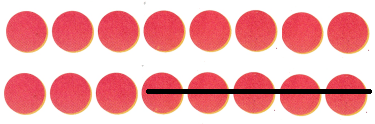Share and Show Math Board

Question 1.
Use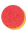to model the problem. Draw the. Gwen has 18 dolls. She gets 2 more. Then she gives 6 dolls to Mae. How many dolls does Gwen have?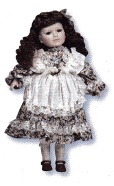_____ dolls
Tell a friend how you chose a ____ strategy to solve the problem.Explanation:
18 + 2 = 20
20 – 6 = 14
Gwen have 14 dolls

Problem Solving

Useto model the problem. Draw the.

Question 2.
H.O.T. Multi-Step Meg has a bowl of grapes. 7 grapes are red. 6 grapes are green. She eats all the green grapes. She eats 2 red grapes. How many grapes are left in the bowl?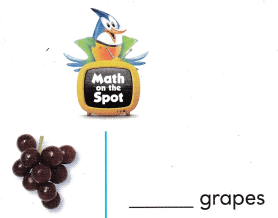Explanation:
7 + 6 = 13
7 + 2 = 9
13 – 9 = 4 grapes are left in the bowl

Question 3.
H.O.T. Multi-Step Ellen puts peaches in a box. Then she gives 6 to Juan. She eats 1 peach. There are 8 peaches left in the box. How many peaches did Ellen start with?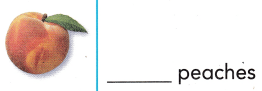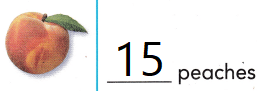Explanation:
6 + 1 = 7
8 + 7 = 15

Question 4.
Multi-Step Choose a number between 10 and 20 to show the number of oranges Rick has. Then solve. Rick has ______ oranges. He gives 3 to Maria. He gives 5 to Paul. How many oranges does Rick have? How many oranges does Rick give away?
3 + 5 = ____
How many oranges does Rick have now?
___ – ___ = ____The number between 10 and 20 so, chose 15
Now rick has 15 oranges
3 + 5 = 8
15 – 8 = 7

Question 5.
Alex finds 4 berries. Alice finds 5 berries. They take the berries home. They eat 3 berries. How many berries are there now?(A) 6
(B) 2
(C) 9
Explanation:
Alex finds 4 berries. Alice finds 5 berries.
4 + 5 = 9
They take the berries home. They eat 3 berries.
9 – 3 = 6

Question 6.
Use Tools There are 10 birds in a tree. 2 birds are blue. The rest are yellow. 1 yellow bird flies away. How many yellow birds are in the tree now?(A) 9
(B) 8
(C) 7
Explanation:
There are total 10 birds,2 birds are Blue
10-2=8
8 are yellow,1 bird flew away so,
8-1=7

Question 7.
Maddy gave 3 small cars to her brother. How many cars does Maddy have now?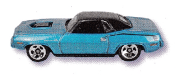(A) 15
(B) 9
(C) 12
Explanation:
Maddy has 5 big cars and 7 small cars
5+7=12
she gave 3 small cars to her brother
5+4=9

Question 8.
Texas Test Prep Kendra has 4 brown rocks and 10 gray rocks. She gives 4 rocks to Amy. How many rocks does Kendra have?
(A) 14
(B) 7
(C) 10
Explanation:
Kendra has 4 brown rocks and 10 gray rocks
4+10=14
she gave 4 rocks to Amy
10-4=10

Take Home Activity

### Texas Go Math Grade 1 Lesson 8.4 Homework and Practice Answer Key

Drawto solve.

Question 1.
Multi-Step Ben has some apples. 9 are red apples. 7 are yellow apples. He gives all the yellow apples to Andy. He eats 1 red apple. How many apples does he have left?
__ apples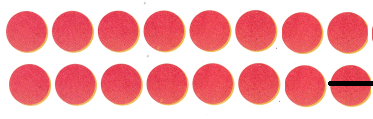Explanation:
Ben has some apples. 9 are red apples. 7 are yellow apples.
9 + 7 = 16
He gives all the yellow apples to Andy. He eats 1 red apple.
16 – 15 = 1

Problem Solving

Drawsto solve.

Question 2.
Multi-Step Choose a number between 10 and 18 to show the number of toy animals Marcy has. Then solve.
Marcy has ____ toy animals. She gives 6 to Leslie. She gives 4 to Beth.
How many toy animals does Marcy give away?
6 + 4 = ___
How many toy animals does Marcy have now?
___ – ___ = ___
____ toy animals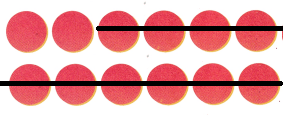2 toy animals
Explanation:
Marcy has 12 toy animals ,she gave 6 to Leslie and 4 to Beth
6+4=10
12-10=2
Marcy has 2 toy animals.

Texas Test Pre

Question 3.
Multi-Step Peter caught 4- fish. Edie caught 7 fish. Edie put 4- fish back in the pond. How many fish do they have now?
(A) 7
(B) 11
(C) 4
Explanation: peter caught 4 fish and caught 7 fish ,
Edie put 4 fish back in the bond 7-4=3
so now Edie has 3 fish and peter has 4 fish
3+4=7

Question 4.
Multi-Step Mike sees 12 frogs in a pond. 3 frogs are green. The rest are brown. 3 brown frogs hop away. How many brown frogs do they see in the pond now?
(A) 9
(B) 12
(C) 6
Explanation: mike sees 12 sees frogs in a pond , 3 frogs are green
12-3=9
9 frogs are brown,3 brown frogs hop away
9-3=6

Question 5.
Multi-Step Derek has 11 stickers. 6 stickers are car stickers. The rest are animal stickers. Derek buys 3 more animal stickers. How many animal stickers does Derek have now?
(A) 9
(B) 8
(C) 11
Explanation:
Derek has 11 stickers,6 stickers are car stickers
11-6=5
5 are animal stickers, He adds 3 more animal stickers
5+3=3

Question 6.
Multi-Step Laura has 8 red pencils and 6 green pencils. She gives 7 pencils to Dwayne. How many pencils does Laura have now? Write the number sentences. Explain the strategy you used to solve the problem.